Courses

# Test: Set Theory- 3

## 15 Questions MCQ Test Quantitative Aptitude (Quant) | Test: Set Theory- 3

Description
This mock test of Test: Set Theory- 3 for CAT helps you for every CAT entrance exam. This contains 15 Multiple Choice Questions for CAT Test: Set Theory- 3 (mcq) to study with solutions a complete question bank. The solved questions answers in this Test: Set Theory- 3 quiz give you a good mix of easy questions and tough questions. CAT students definitely take this Test: Set Theory- 3 exercise for a better result in the exam. You can find other Test: Set Theory- 3 extra questions, long questions & short questions for CAT on EduRev as well by searching above.
QUESTION: 1

### A survey on a sample of 25 new cars being sold at a local auto dealer was conducted to see which of the three popular options—air conditioning, radio and power windows—were already installed. The survey found: 15 had air conditioning 2 had air conditioning and power windows but no radios 12 had radio 6 had air conditioning and radio but no power windows 11 had power windows 4 had radio and power windows 3 had all three options What is the number of cars that had none of the options?

Solution:

From the given conditions, we have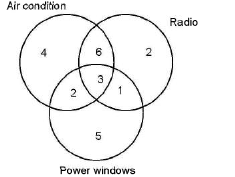When we add all the values, we get a total of 23 cars.

So, 2 cars don’t have the air conditioning or the radio, or the power windows.

QUESTION: 2

### How many numbers from 1 to 100 are not divisible by either 2 or 4 or 5?

Solution:

Let us first understand the meaning of the statement given in the question—

It is given that the numbers are from 1 to 100, so while counting we will include both the limits, i.e., 1 and 100. Had this been “How many numbers in between 1 to 100 are..." then we would not have included either 1 or 100.

Now to solve this question, we will first find out the number of numbers from 1 to 100 which are divisible by either 2 or 5 (since all the numbers which are not divisible by 2 will not be divisible by 4 also, so we do not need to find the numbers divisible by 4). And then we will subtract this from the total number of numbers i.e., 100. It can be seen below:

Total number of numbers = numbers which are divisible + numbers which are not divisible

So, n(2U5) = n(2) + n(5) - n (2∩5)

Now, n(2) = 50

n(5) = 20

n(2∩5) = 10

n(2U5) = 50 + 20 - 10 = 60

Numbers which are not divisible = total number of numbers-numbers which are divisible

=100 - 60 = 40

QUESTION: 3

### In a class of 50 students, 70% students pass in QA and 60% pass in RC. What is the minimum percentage of students who pass in both the papers?

Solution:

We know that n (A∪B ) = n(A ) + n(B) - n(A∩B)

Assuming that n(Q) = number of students passing QA and n(R) = number of students passing RC.

Hence, n(Q∪R ) = n(Q) + n(R) - n(Q∩R)

Or, n(Q∩R) = n(Q) + n(R) - n(Q∪R) = 70% + 60% - n(Q∪R)

Now the minimum value of n(Q∩R) will occur for the maximum value of n(Q∪R).

The maximum possible value of n(Q∪R) = 100%

So, the minimum value of n(Q∩R) = 30%

QUESTION: 4

In a class of 50 students, 70% students pass in QA, 90% pass in EU, 85% pass in DI and 60% pass in RC. What is the minimum percentage of students who pass in all the papers?

Solution:

This question is just the extension of the earlier question.

The minimum value of EU and (QA∩RC) = 90% + 30% - 100% = 20%

The minimum value of DI and EU and  (QA∩RC) = 20% +85% - 100% = 5%

QUESTION: 5

In a class of 50 students, 70% students pass in QA, 90% pass in EU and 60% pass in RC. What is the minimum percentage of students who pass in all the papers?

Solution:

We can do this question by using either the formula for three sets, or we can simply keep on applying the formula for two sets required a number of times.

We know that n(A∪B) = n (A ) + n(B) - n(A∩B)

Assuming that n(Q) = number of students passing QA, n(E) = number of students passing EU and n(R) = number of students passing RC

Hence, n(Q∪R) = n(Q) + n(R) - n(Q∩R).

Or, n(Q∩R) = n(Q) + n(R) - n(QuR) = 70% + 60% - n(Q∪R)

Now the minimum value of n(Q∩R) will occur for the maximum value of n(Q∪R). The maximum possible value of n(Q∪R) =100%

So, the minimum value of n(Q∩R) = 30%

Now, the minimum value of EU, QA and RC will be obtained by finding the minimum of EU and (QA∩RC).

The minimum value of EU and (QA∩RC) = 90% + 30% - 100% = 20%

QUESTION: 6

At    the    birthday    party    of    Sherry,    a    baby    boy,    40    persons    chose    to    kiss   him    and    25    chose    to    shake    hands with    him.    10    persons    chose    to    both   kiss    him    and    shake    hands    with    him.    How    many    persons    turned    out    at the    party?

Solution: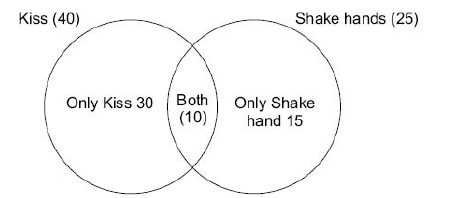From the figure, it is clear that the number of  people at  the party were 30+10+15 = 55.

we can of course solve this mathematically as below:

Let  n(A) = No. of  persons    who kissed Sherry = 40

n(B) = No. of  persons  who    shake hands with Sherry  =  25

and n(A « B) = No. of   persons who shook  hands    with Sherry and kissed him    both =10

Then using  the formula, n(A    »B) = n(A) + n(B) -n(A « B)

n(A »B) =40 + 25  -10 = 55

QUESTION: 7

In the Mindworkzz club all the members participate either in the Tambola or the Fete. 320 participate in the Fete, 350 participate in the Tambola and 220 participate in both. How many members does the club have?

Solution: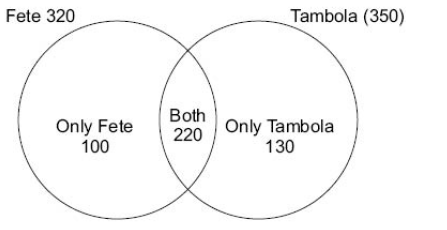The total number of peoples = 100 + 220+130 = 450

So, Option D is correct

QUESTION: 8

There are 20000 people living in Defence colony,  Gurgaon. Out  of  them 9000  subscribe  to Star TV Network and 12000 to Zee TV Network. If  4000 subscribe to both,how many do not  subscribe  to any of the  two?

Solution: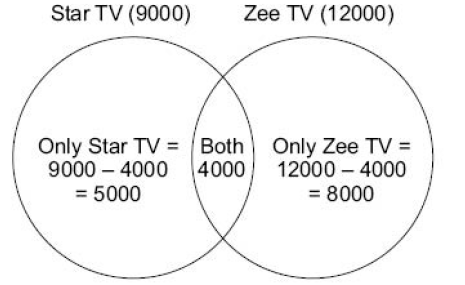The required answer would be = 20000 - 4000 - 5000 - 8000 = 3000

QUESTION: 9

In a group of 120  athletes, the number of athletes who can  play Tennis, Badminton, Squash and Table Tennis is 70, 50, 60 and 30 respectively. What is the maximum number   of athletes who can play none of the games

Solution:

In order to think of the maximum   number of  athletes who can play   none of  the games, we can think   of  the fact that since there  are 70 athletes who play tennis, fundamentally there are a maximum of 50 athletes who would be possibly in the can play none of the games’.

No other constraint in the problem necessitates a reduction of this number and hence the answer to this question is 50.

QUESTION: 10

Directions for Questions 1 to 3:

Refer to the data  below and answer  the questions that  follow.

Last year, there were 3 sections in the Catalyst, a mock CAT paper. Out of them 33  students cleared the cut-off in Section 1, 34 students cleared the cut-off in Section 2 and 32 cleared the cut-off in Section 3. 10 students cleared the cut-off in Section 1 and Section 2, 9 cleared the cut-off in Section 2 and Section 3, 8 cleared the cut-off in Section 1 and Section 3. The number of people who cleared each section alone wasequal and was 21 for each section.

How many cleared all the three sections?

Solution: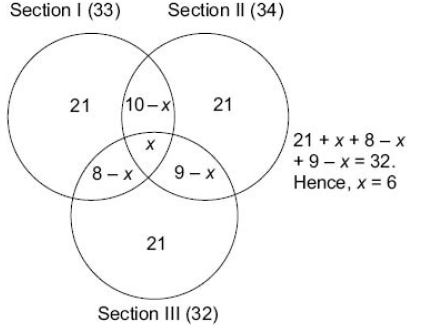since x = 6 , the figure becomes: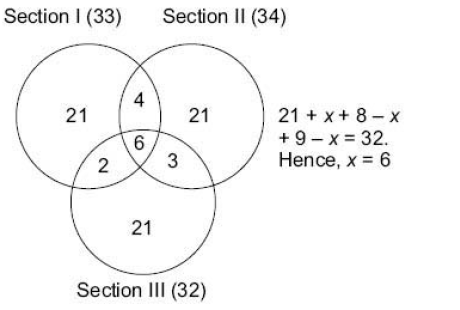6. option (B) is correct

QUESTION: 11

Last year, there were 3 sections in the Catalyst, a mock CAT paper. Out of them 33  students cleared the cut-off in Section 1, 34 students cleared the cut-off in Section 2 and 32 cleared the cut-off in Section 3. 10 students cleared the cut-off in Section 1 and Section 2, 9 cleared the cut-off in Section 2 and Section 3, 8 cleared the cut-off in Section 1 and Section 3. The numberof people who cleared each section alone wasequal and was 21 for each section.

How many cleared only one of the three sections ?

Solution:

21 + 21 +21 = 63

Option (B) is correct

QUESTION: 12

Last year, there were 3 sections in the Catalyst, a mock CAT paper. Out of them 33  students cleared the cut-off in Section 1, 34 students cleared the cut-off in Section 2 and 32 cleared the cut-off in Section 3. 10 students cleared the cut-off in Section 1 and Section 2, 9 cleared the cut-off in Section 2 and Section 3, 8 cleared the cut-off in Section 1 and Section 3. The numberof people who cleared each section alone wasequal and was 21 for each section.

The ratio of  the number of students clearing then cut-off in one or  more of  the sections to     the number of  students clearing the cutoff  in Section 1 alone is?

Solution:

(21+ 21 +21 +6+4+3+2)/21 = 78/21

Option (A) is correct

QUESTION: 13

Directions:

In a locality having 1500  households, 1000 watch Zee TV, 300 watch NDTV and 750 watch Star Plus.
Based on this information answer the questions that follow:

The minimum number of households watching Zee TV and Star Plus is:

Solution:

If we try to consider each of the households watching Zee TV and   StarPlus as independent of  each   other, we would get a total of 1000 + 750 = 1750 households.However, wehave a totalof only1500 householdsi the locality and hence, there has to be a minimum interference of atleast 250 households whowould  be watching both Zee TV and Star Plus. Hence, the answer to this question is 250.

QUESTION: 14

Directions:

In a locality having 1500  households, 1000 watch Zee TV, 300 watch NDTV and 750 watch Star Plus.
Based on this information answer the questions that follow:

The minimum number of  households watching both Zee TV and NDTV is:

Solution:

In this case,the number of households watching ZeeTV and NDTV can be separate from each other since there is no interference required between the households     watching ZeeTV and the households watching NDTV  as their individual sum(1000 +300) is smaller than the 1500 available householdsin the locality.Hence,the answer in this question is 0.

QUESTION: 15

Directions:

In a locality having 1500  households, 1000 watch Zee TV, 300 watch NDTV and 750 watch Star Plus.
Based on this information answer the questions that follow:

The maximum number of  households who watch neither of the three channels is:

Solution:

For this  to occur  the following situation would give us the required solution: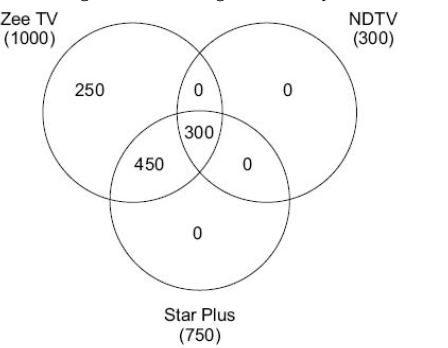As you can clearly see from the figure, all the requirements of each category of viewers is fulfilled by the given allocation of 1000 households. In this situation,the maximum number of households who do not watch any of the three channels is visible as 1500 –1000 = 500.